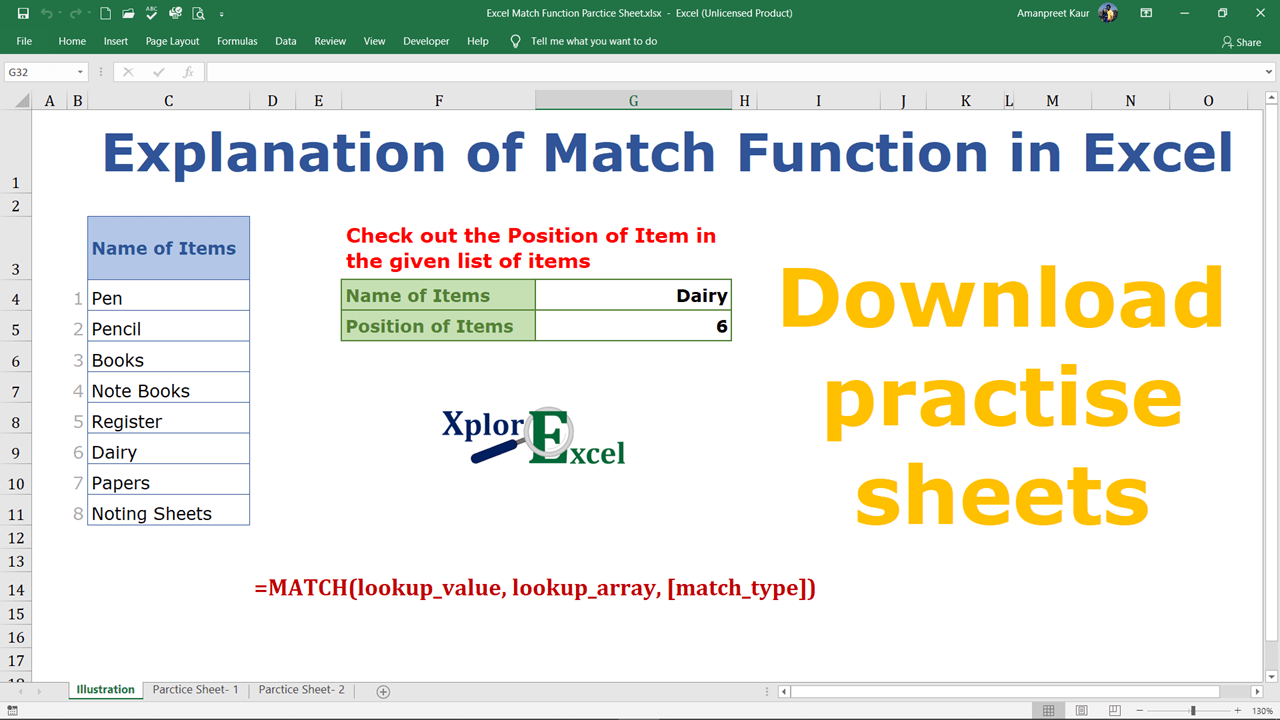## Excel Match Function:-

The Excel Match function is used to determine the selected value position in the selected array. The position of the selected value may be exactly matched, less than, and more than from the value.

### The feature of the “Match” function: –

• With the help of the Match Function, we can retrieve the position of the Selected value in the selected array and use this position further with the index function and others.
• It helps in getting a particular position of a certain value in the selected array.

### Explanation of Function:-

Now, We will explain the Arguments of the Function.

`=MATCH(lookup_value, lookup_array, [match_type])`

lookup_value: –

The lookup_value the value of which we want to know the position in the selected array.

lookup_array: –

The lookup_array selected cells from which we want to know the position of the lookup_value.

match_type: –

The match_type are of three types show as following: –

1.  If we put the value “1”  – The MATCH function will find the largest value that is less than or equal to the value. You should be sure to sort your array in ascending order.
2. If we put the value “0”  –  The MATCH function will find the first value that is equal to the value. The array can be sorted in any order.
3. If we put the value “-1” – The MATCH function will find the smallest value that is greater than or equal to the value. You should be sure to sort your array in descending order.

### Example of Function: –

I will show you, how to apply the whole function. (using optional also).

From the following table get the position of the papers in the selected array: –

Solution:-

We will Apply the Match function and get the result. This is shown in the following steps: –

Step No. 1:-

We will write the “=Match( ” in the column of the result.

Step No. 2

Now select the cells with which you want to match the value with the selected array.

Step No. 3

Now select the array.

Step No. 4

Now apply the matching condition

Step No. 5: –

Now, Copy and paste the function in all remaining cells to get the result.

Thanks

Also, Check out the following function of excel: –

1. #### Excel SUMIF Function - Download practice Sheet - Xplore Excel

April 26, 2021

2. #### COUNT Function in Excel- Download 2 Practice Sheet - Xplore Excel

April 26, 2021

3. #### Sum Function in Excel - Download 2 Practice Sheets - Xplore Excel

April 26, 2021

4.#### zortilonrel

April 26, 2021

What¦s Going down i’m new to this, I stumbled upon this I’ve found It positively useful and it has helped me out loads. I am hoping to give a contribution & aid different users like its aided me. Good job.

5. #### IF Function in Excel - Free Explanation with example - Xplore Excel

April 26, 2021

6. #### IFERROR Excel Function- Free 2 Practice workbooks

April 26, 2021

7. #### IFNA Excel Function | Free 2 Practice workbooks - Xplore Excel

April 26, 2021

8.#### Xavier B. J. Dsouza

April 26, 2021

Thank you very much. You all are doing an excellent job. I am just a Newbie here for learning Excel. Thank you once again. I am interested in taking Practical lessons with Templates to learn and study in theory and practice.
However, I was searching for such a Website and I came across yours.
Xavier B. J. Dsouza

1.#### Sarbjit Singh

April 26, 2021

Thanks alot.

2.#### Sarbjit Singh

April 26, 2021

Thanks a lot.